Open in App
Not now

# How to change figure size in Plotly in Python

• Last Updated : 23 Jan, 2023

In this article, we will discuss how we can change the figure size in Plotly in Python. Let’s firstly take about Plotly in Python.

### Plotly

Python library provides us with an interactive open-source Plotly library that can support over 40 unique chart types that cover a wide list of statistical, financial, geographic, scientific, and 3-dimensional use cases.

To install the plotly, run the below command in the terminal:

`pip3 install plotly.express`

Once we installed the library, we can now change the figure size by manipulating the graph size and the margin dimensions. It can also be achieved using different methods for different use cases.

### Changing Height, Width, & Margins with Plotly Express

We will now try to change the figure size by modifying the width and heights using Plotly express. One may use it to plot the same interactive charts with few lines of code.

Let’s see an example, where we have first imported the plotly.express library. Then we will use data.wind() method we are creating a data frame, then scatterplot the dataframe.

## Python

 `# Importing plotly library``import` `plotly.express as plotly` `# Create a dataframe``dataFrame ``=` `plotly.data.wind()` `# Plot the figure``myFigure ``=` `plotly.scatter(dataFrame, x``=``"direction"``, y``=``"frequency"``,``                 ``width``=``400``, height``=``400``)` `# Display the figure``myFigure.show()`

Output: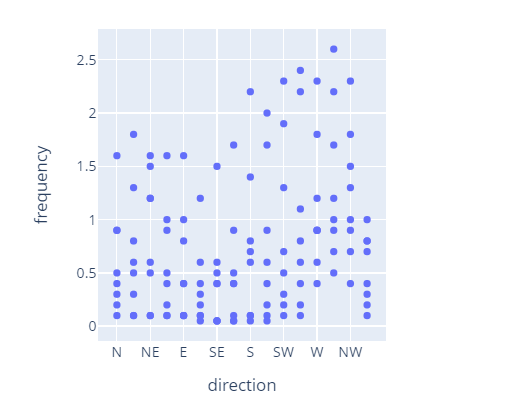Let’s see an example where we can update the margins of the graph using margin dimensions, right top, left, and bottom (l, r, t, b). And these margin parameters have been passed to the update_layout() method that is called on the figure object. By applying this, we have finally constructed the graph objects in the chart area to give a better visualization.

## Python3

 `# Importing plotly.express library``import` `plotly.express as plotly` `# Creating a dataframe``dataframe ``=` `plotly.data.tips()` `# Creating a figure using scatter() method``myFigure ``=` `plotly.scatter(dataframe, x``=``"total_bill"``,``                          ``y``=``"tip"``, facet_col``=``"sex"``,``                          ``width``=``600``, height``=``400``)` `# Modifying the figure layout update_layout() method``myFigure.update_layout(``    ``margin``=``dict``(l``=``30``, r``=``30``, t``=``30``, b``=``20``),``    ``paper_bgcolor``=``"LightSteelBlue"``,``)` `# Display the figure to console``myFigure.show()`

Output: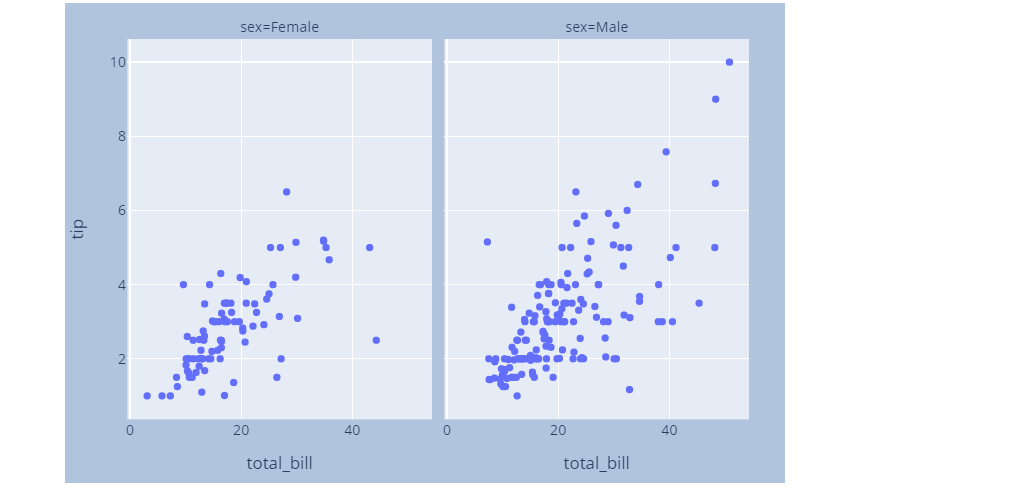### Change Height, Width, & Margins with Graph Objects in plotly

Now we will use graph objects instead of plotly express in order to change the height, width, and margins. To have greater control over chart elements one may use graph objects instead of plotly express. Graph objects are considered building blocks of figures at the low level.

## Python3

 `# Importing plotly.graph_objects and plotly.express``import` `plotly.graph_objects as plotly``import` `plotly.express as px` `# Generating a figure``myFigure ``=` `plotly.Figure()` `# Creating a dataframe``dataFrame ``=` `px.data.wind()` `# Add traces to the figure``myFigure.add_trace(plotly.Scatter(``    ``x``=``dataFrame[``"direction"``],``    ``y``=``dataFrame[``"frequency"``]``))` `# Display the figure``myFigure.show()`

Output: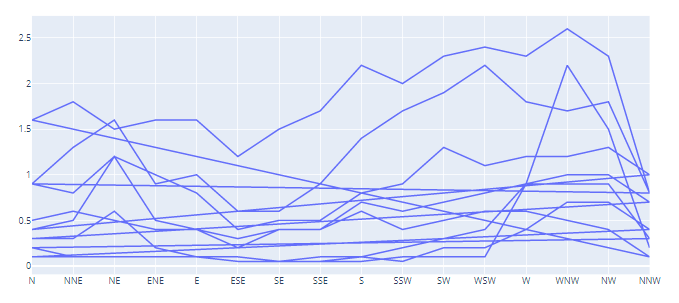In this example, in order to set the chart area we have used height and width. The margin parameters are used to set the graph objects. Note that this time all these arguments have been used inside the update_layout() function of the figure object.

## Python3

 `# Importing plotly.graph_objects and plotly.express``import` `plotly.graph_objects as plotly``import` `plotly.express as px` `# Generating a figure``myFigure ``=` `plotly.Figure()` `# Creating a dataframe``dataFrame ``=` `px.data.wind()` `# Adding traces to the figure``myFigure.add_trace(plotly.Scatter(``    ``x``=` `dataFrame[``"direction"``],``    ``y``=` `dataFrame[``"frequency"``]``))`  `# Lets update the figure using update_layout() method on myFigure``myFigure.update_layout(``    ``title``=``"Wind Frequencies"``,``    ``xaxis_title``=``"Direction"``,``    ``yaxis_title``=``"Frequency"``,``    ``autosize``=``False``,``    ``width``=``400``,``    ``height``=``500``,``    ``margin``=``dict``(l``=``30``,r``=``30``,b``=``30``,``                ``t``=``30``,pad``=``3``                ``),``    ``paper_bgcolor``=``"LightSteelBlue"``,``)` `# Display the figure``myFigure.show()`

Output: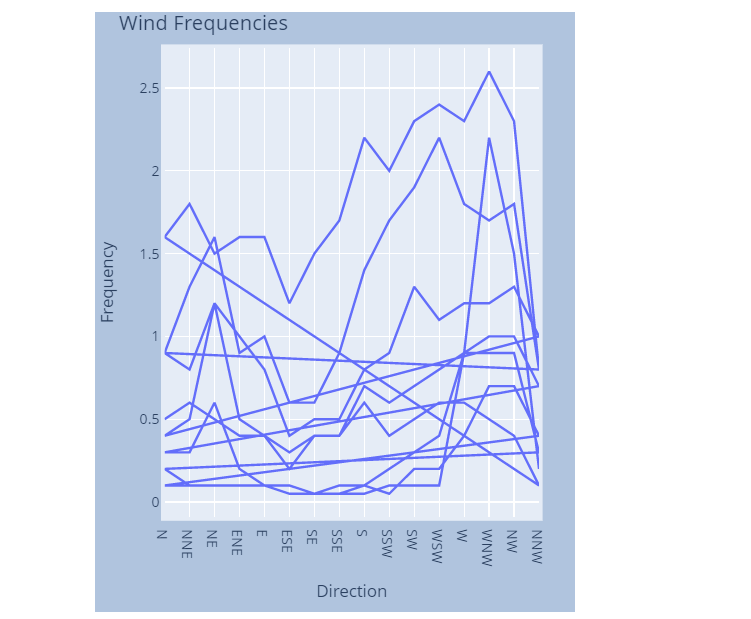### Automating margin changes in plotly

Now we will try to automate margin changes in plotly.

## Python3

 `# Importing plotly.graph_objects and plotly.express``import` `plotly.graph_objects as plotly``import` `plotly.express as px` `myFigure ``=` `plotly.Figure()` `myFigure.add_trace(plotly.Bar(``    ``x``=``[``"Moranp"``, ``"Turquoise"``, ``"Cornflower"``, ``"Raddles"``],``    ``y``=``[``6``, ``4``, ``5``, ``11``]``))` `myFigure.update_layout(``    ``autosize``=``False``,``    ``width``=``700``,``    ``height``=``700``,``    ``yaxis``=``dict``(``        ``title_text``=``"Heights"``,``        ``ticktext``=``[``"little"``, ``"very long title"``, ``"long"``, ``"short title"``],``        ``tickvals``=``[``2``, ``4``, ``6``, ``8``],``        ``tickmode``=``"array"``,``        ``titlefont``=``dict``(size``=``20``),``    ``)``)` `myFigure.update_yaxes(automargin``=``False``)` `myFigure.show()`

Output: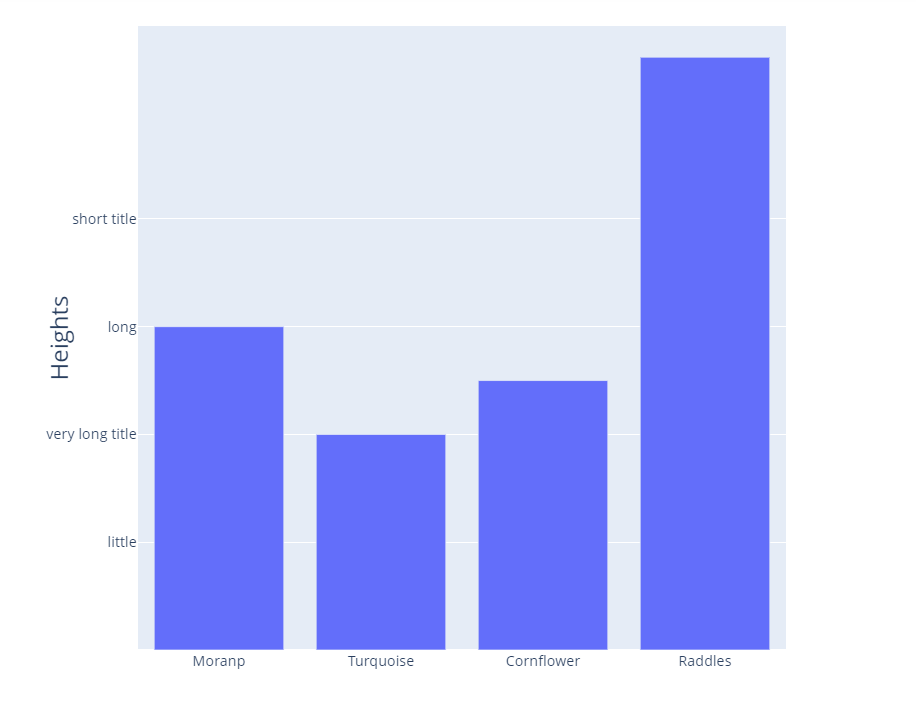As you can see above without using margin manipulation, the y-axis tick labels can overlap with the y-axis title.

To avoid this overlapping, we are required to set “automargin” as True. By doing so, it will automatically adjust the margin size. And the automargin argument would resist tick labels from overlapping with the axis titles.

## Python3

 `myFigure.update_yaxes(automargin``=``True``)` `myFigure.show()`

Output: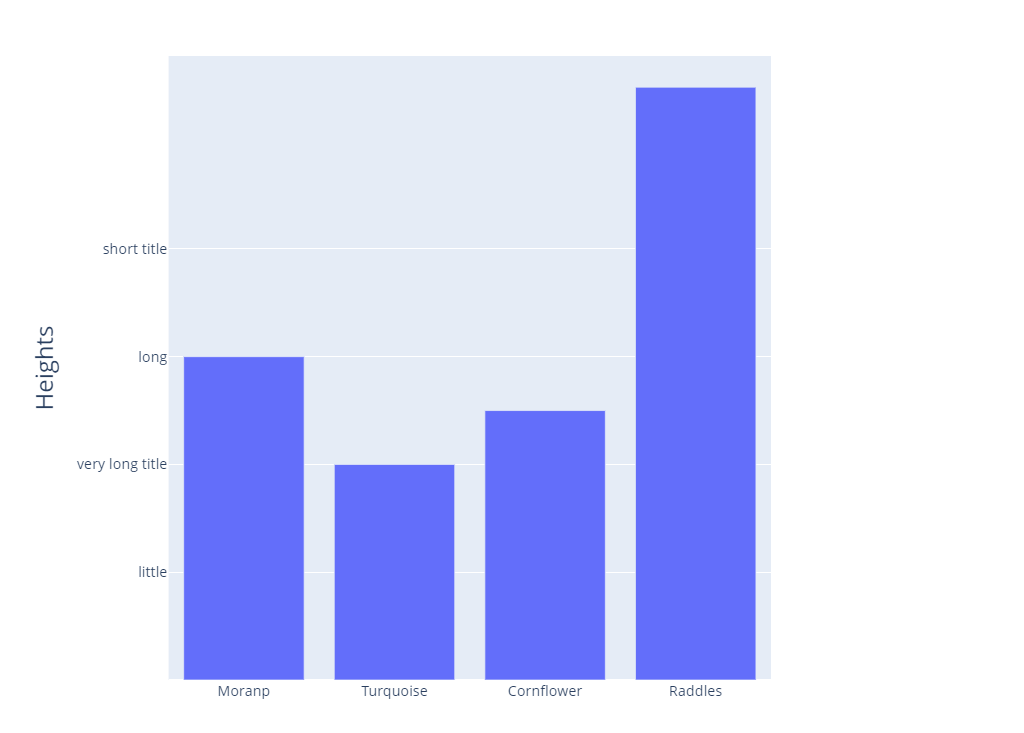My Personal Notes arrow_drop_up